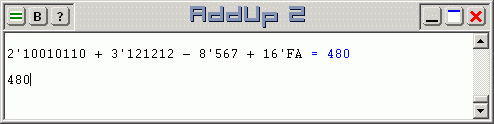# Numeric Base Operations with AddUp 2

AddUp reads numbers expressed in any number base from 2 to 36. Expressing a number in a numeric base other than 10 are done by specifying a base prefix for the number. This prefix consists of the base value plus a single quote. Base-2 (binary) numbers use a 2' prefix, base-3 numbers use a 3' prefix, base-4 numbers use a 4' prefix, and so on. For base-10 (decimal) numbers, this could mean using a 10' prefix before the number; this is redundant since base-10 is assumed unless a different one is specified, but it is allowed.

Here are examples of numbers expressed using various number bases in AddUp 2.

• Binary (base-2) number 2'10010110 represents decimal value 150.
• Base-3 number 3'121212 represents decimal value 455.
• Octal (base-8) number 8'567 represents decimal value 375.
• Hexadecimal (base-16) number 16'FA represents decimal value 250.
Calculations can also involve numbers expressed in any number base. It is possible to include numbers expressed in different numeric bases within the same calculation using AddUp's prefix notation. Furthermore, the use of number bases are not restricted to integer values. All numeric formats support all numeric bases: integers, fixed-point, scientific, rational, etc.Since number bases can be mixed at will within an expression, it becomes necessary to determine which base will be used to express the final result. The output of all AddUp calculations is produced in base-10 decimal unless specified otherwise. To show a result in another number base, start an expression with a prefix similar to what is used in front of numbers: start the line with a single quote and then the number of the base. Notice that the quote is on the left side of the requested output base now. This line prefix must be followed by a space to separate it from the expression to evaluate. The expression itself could contain all base-12, or all binary, or all octal numbers, or a mixture of numbers in various numeric bases. But the result will be presented in the number base that is specified by the line prefix. The default base-10 applies if a base is not specified.

Converting numbers from one base to another is easily done. Start the line with a prefix that indicates the desired output base: a single quote, the desired output base value and a separating space. Then write the number that will be converted using any desired numeric base. For example, enter '16 2'10010110 to obtain the hexadecimal (base-16) representation of binary (base-2) number 10010110, which will be expressed as 16'96. You can press Enter again from the result line to convert this hexadecimal value to decimal. Since base-10 is the default, the conversion will be automatic and produce number 150 in normal base-10 if no other base is given.